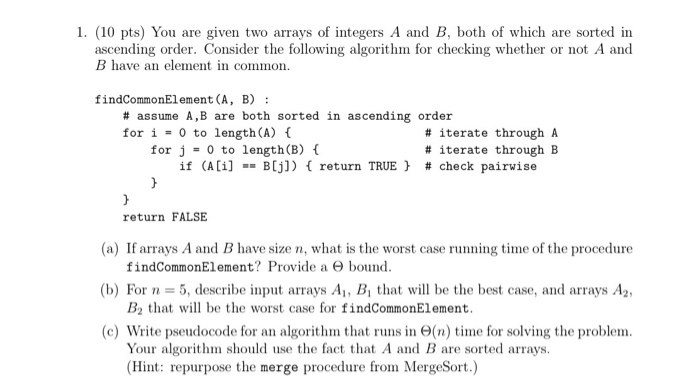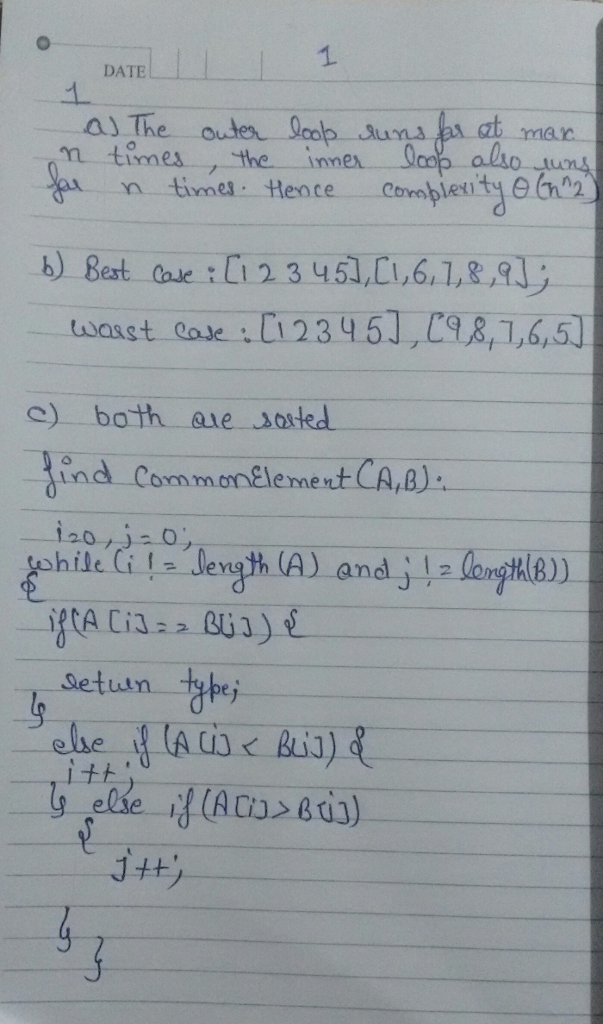# Homework Solution: algorithms…

algorithmsYou are given two arrays of integers A and B, both of which are sorted in ascending order. Consider the following algorithm for checking whether or not A and B have an element in common. findCommonElement(A, B): #assume A, B are both sorted in ascending order for i = 0 to length (A) { # iterate through A for j = 0 to length(B) { # iterate through B if (A[i] == B[j]) {return TRUE} #check pairwise } } return FALSE (a) If arrays A and B have size n, what is the worst case running time of the procedure findCommonElement? Provide a Theta bound. (b) For n = 5, describe input arrays A_1, B_1, that will be the best case, and arrays A_2, B_2 that will be the worst case for findCommonElement. (c) Write pseudocode for an algorithm that runs in Theta(n) time for solving the problem. Your algorithm should use the fact that A and B are sorted arrays.